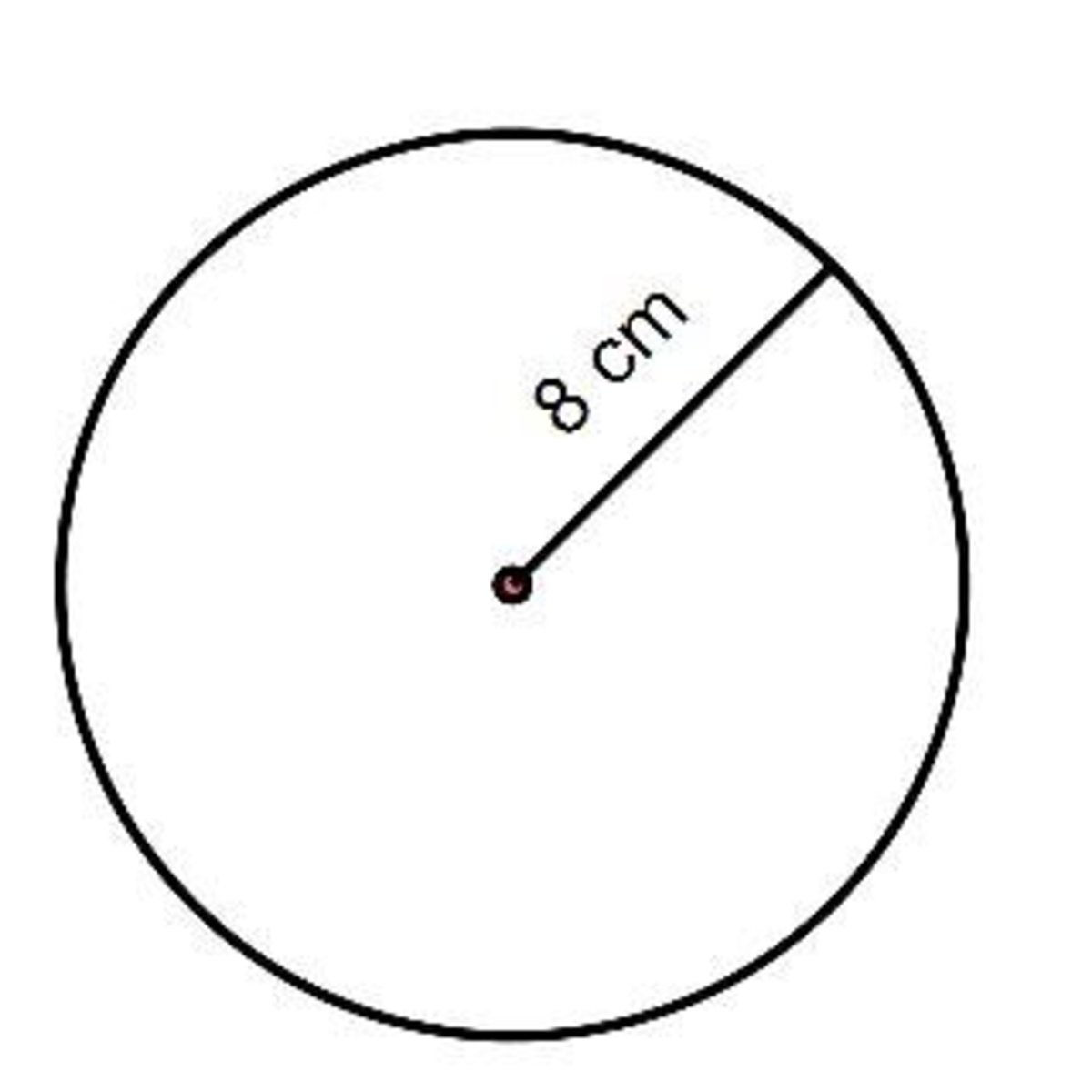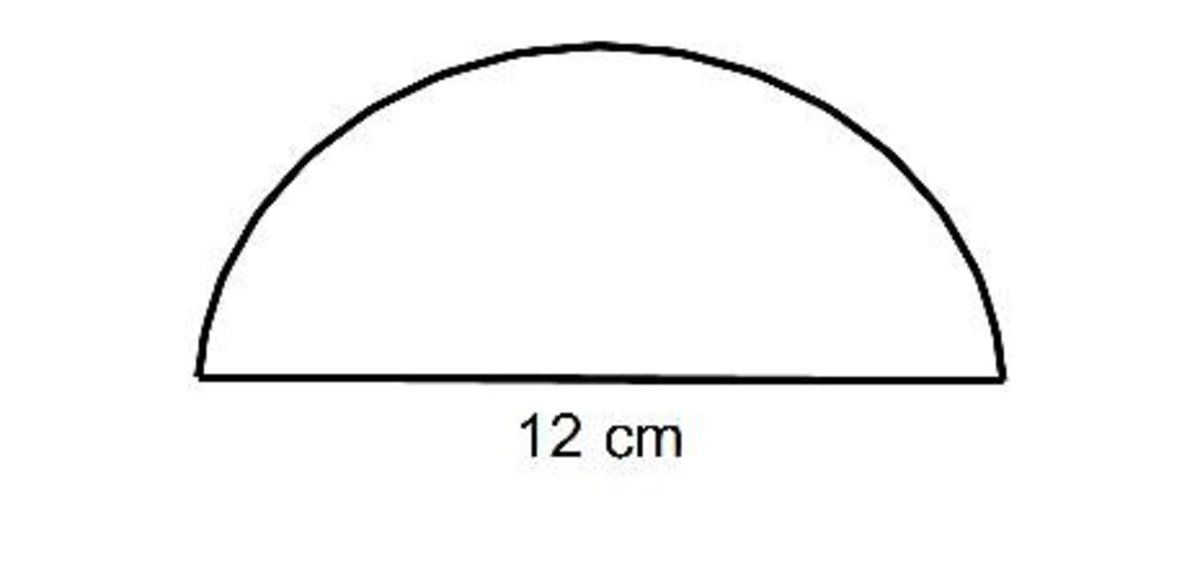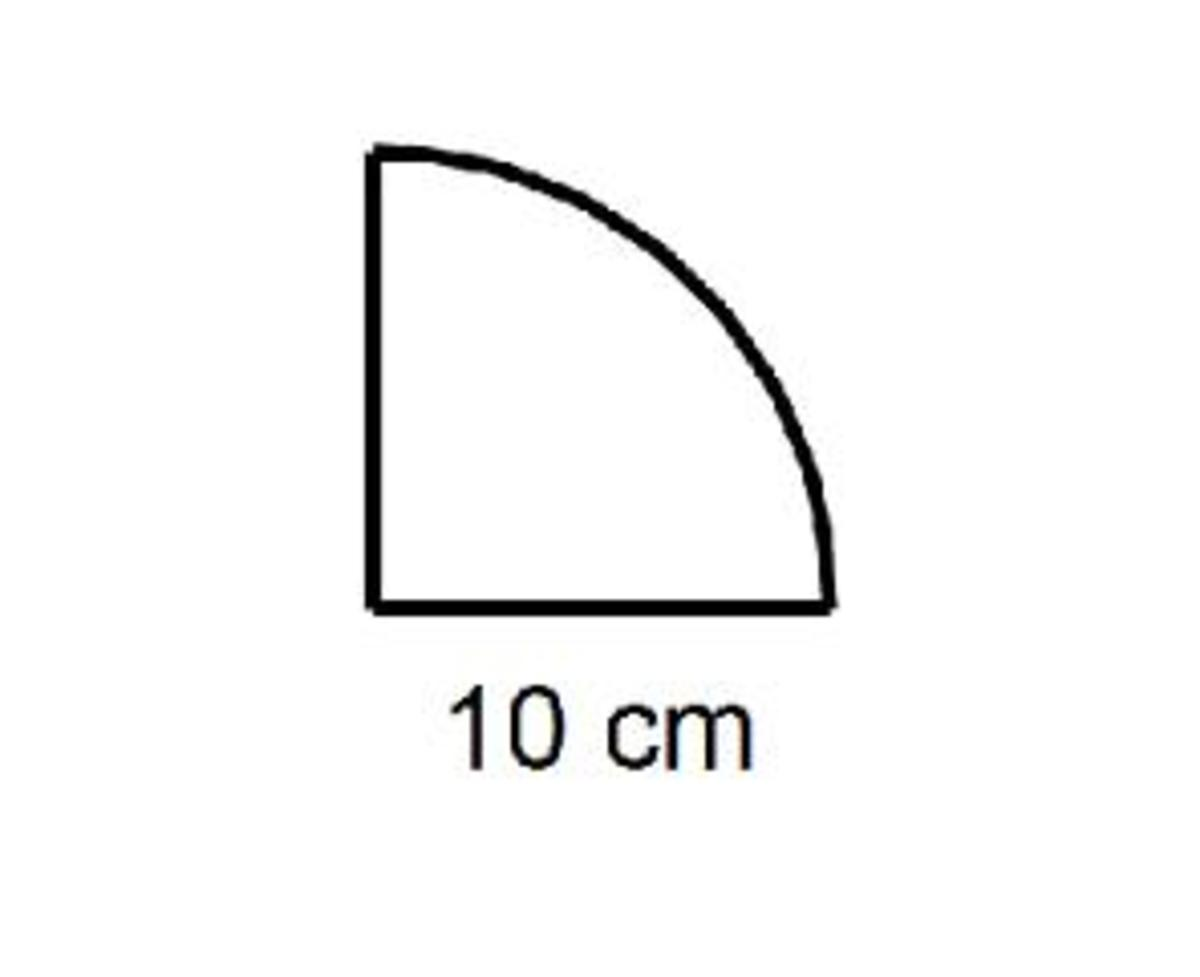Updated date:

# The method for working out the area of a circle, semicircle and a quadrant (math help).

Author:

On this page you will be shown the method for working out the area of a circle, a semicircle (half a circle) and a quadrant (a quarter of a circle).

The area of a circle can be found by using the formula A = πr². The symbol π is Pi and is a number equal to 3.142 rounded to 3 decimal places. So if you need to work out the area of a circle then all you need to do is substitute the radius into this formula. Make sure you substitute the radius into the formula and not the diameter of the circle. The radius is halfway across the circle and the diameter is all of the way across.

Example

Work out the area of this circle.The length given in this question is the radius so you just need to substitute r = 8 into the formula A = πr².

A = πr²

A = 3.142 × 8²

A = 201 cm² rounded to the nearest whole number.

For some more question on the area of a circle make sure you look at this hubpage on circles.

The area of a semicircle can be worked out in a similar way. All you need to do is change the formula to A = ½πr². That’s because a semi-circle takes up half the space as a full circle.

Example

Work out the area of this semicircle.Be careful with this example as the length given in the question is the diameter of the circle. So if this diameter then the radius can be worked out by halving this number. Therefore the radius of our semicircle is 6cm. All you need to do next is substitute this for r in the formula A = ½πr².

A = ½πr².

A = ½ × 3.142 × 6²

A = 57 cm²

If you want some more help on semicircles then make sure you read this page on the area of a semicircle.

Finally, let’s take a look at working out the area of a quadrant. A quadrant is a quarter of a circle so the area of the quadrant can be found by A = ¼ πr². Again, make sure you substitute the radius into the formula.

Example

Work out the area of this quadrant.Here the radius of the circle is given, so r = 10 cm. So all you need to do is substitute this into the formula for the area of a quadrant:

A = ¼πr²

A = ¼ × π × 10²

A = ¼ × 3.142 × 100

A = 79 cm² rounded to the nearest whole number.

So the area of the quadrant shown is 79 cm².

More examples on the area of a quadrant can be found by clicking here.

So to summarise, the method for working out the area of a circle can be done by using the formula πr², the area of a semicircle is ½πr² and the area of quadrant is ¼πr². Just be careful that you substitute the radius into each formula as all formulas require the radius.

## Comments

Sarah Chewings from Nottingham, England on July 04, 2012:

Really clear and well presented, very well done!